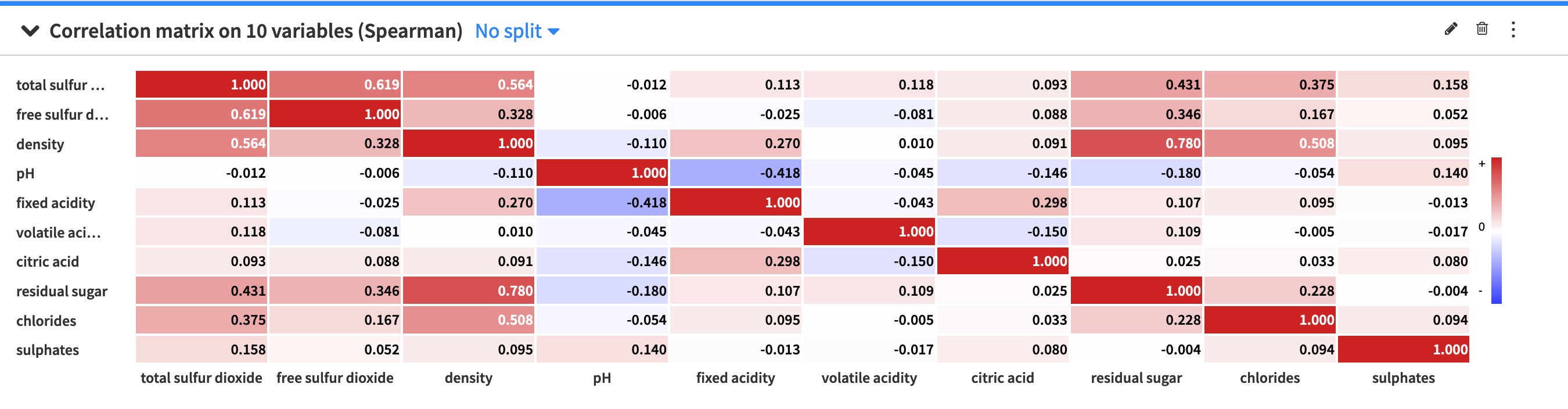# Correlation matrix¶

A correlation matrix is useful for showing the correlation coefficients (or degree of relationship) between variables. The correlation matrix is symmetric, as the correlation between a variable V1 and variable V2 is the same as the correlation between V2 and variable V1. Also, the values on the diagonal are always equal to one, because a variable is always perfectly correlated with itself.

The Correlation matrix card allows you to view a visual table of the pairwise correlations for multiple variables in your dataset. By default, Dataiku DSS computes the Spearman’s rank correlation coefficient, but you can select to compute the Pearson correlation coefficient instead. Note that you can only use numerical variables to compute the correlation matrix.The default setting of the correlation matrix displays signed (positive and negative) correlation values within colored cells, with the colors corresponding to the values. However, you can use the correlation matrix menu (⋮) to configure the visualization of the correlation matrix. The menu provides options to:

• Toggle the values and colors on and off

• Convert correlation values to absolute values

• Set a threshold so that the matrix only displays a correlation value if its magnitude (or absolute value) is greater than the threshold value.